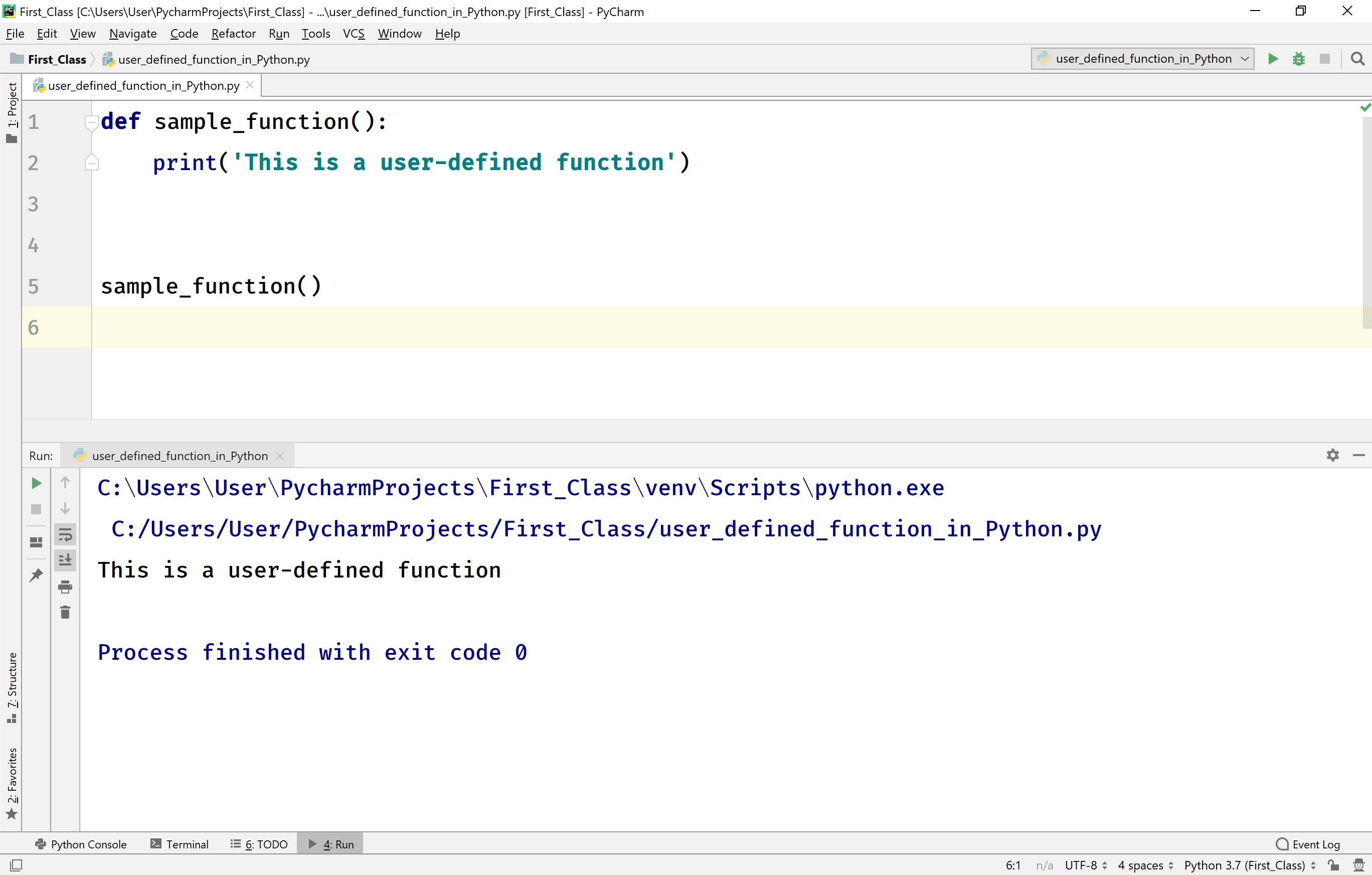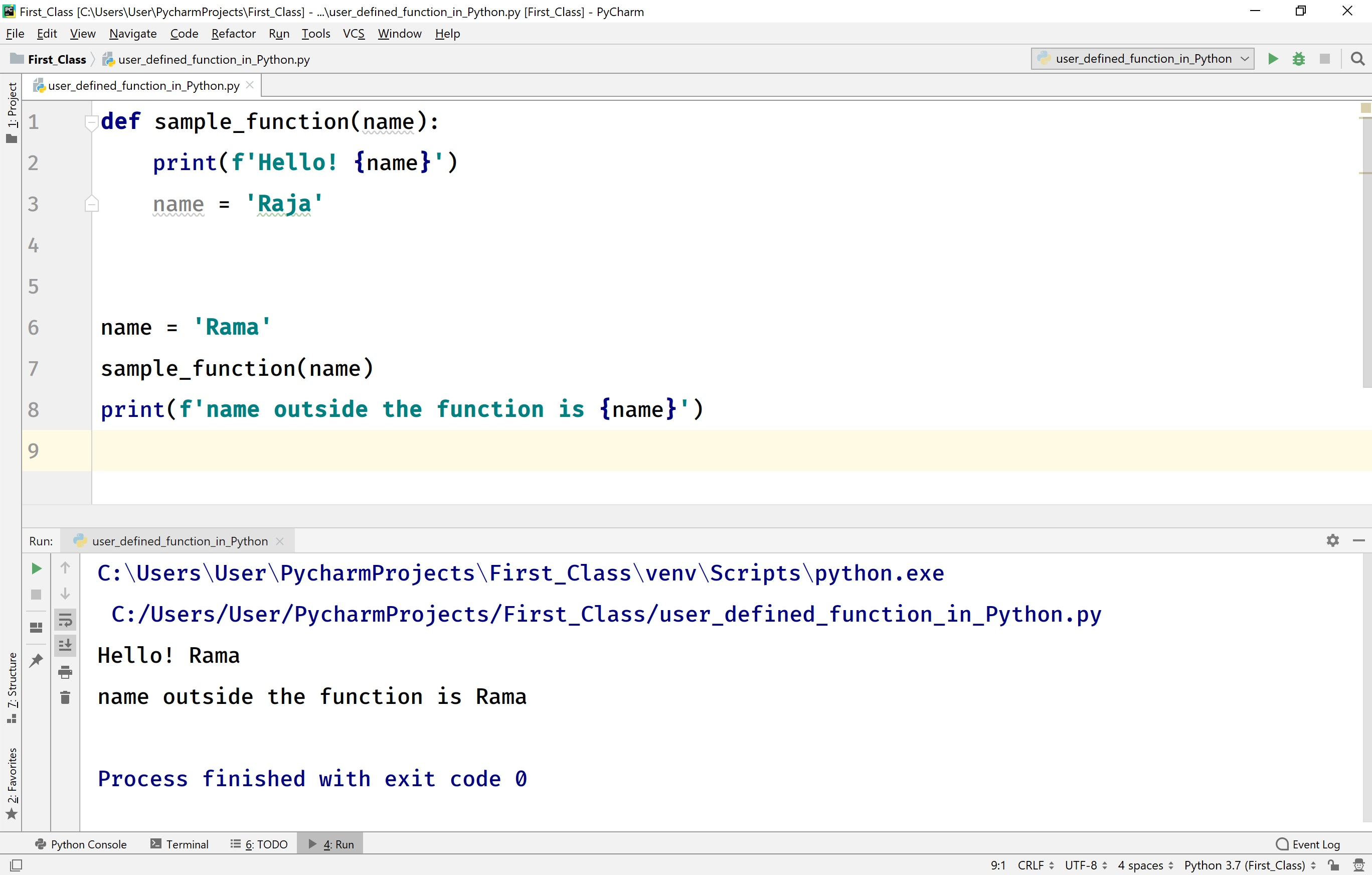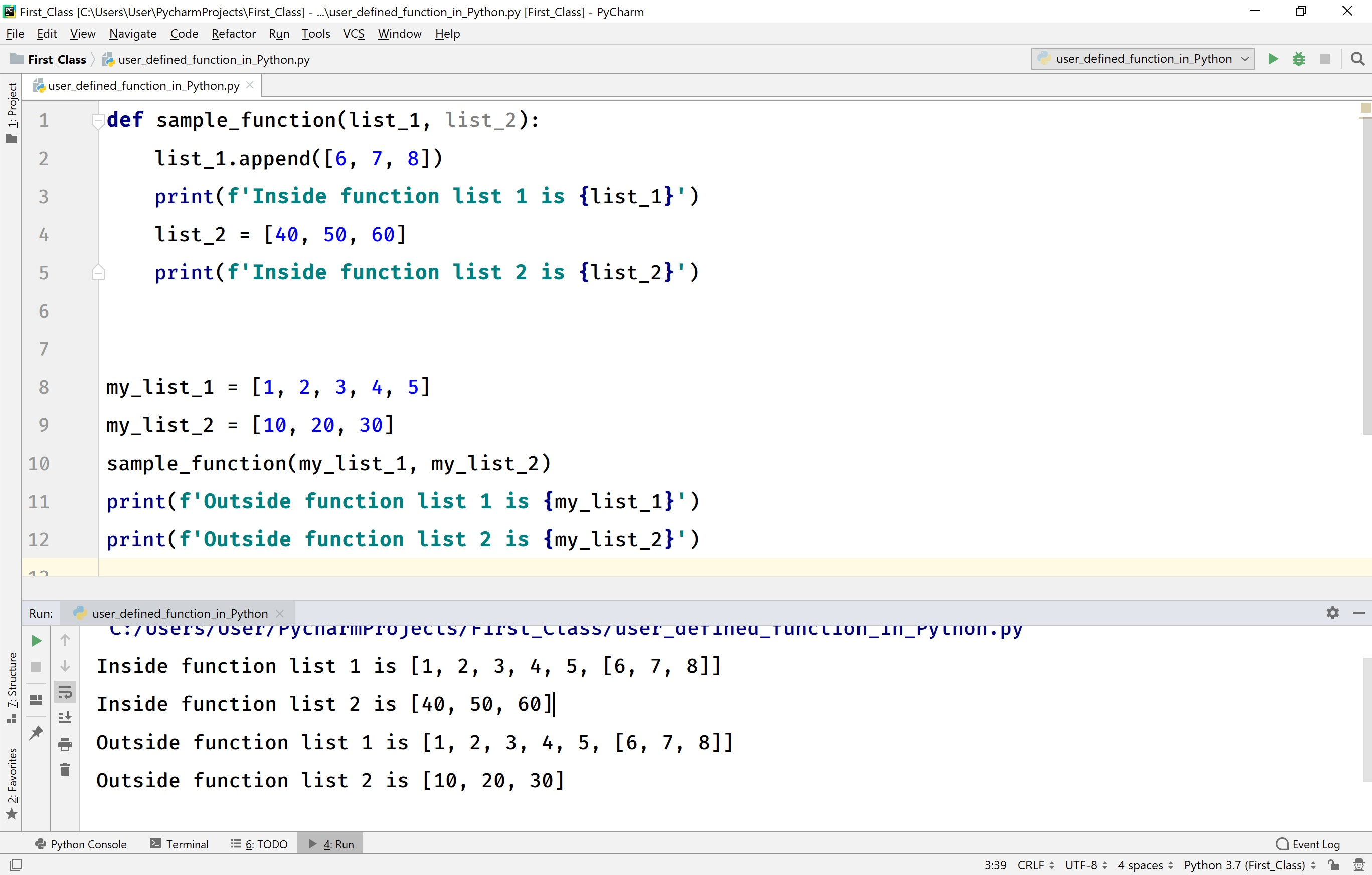The perfect place for easy learning...

×

# Python Functions

A function is a block of statements under a name that can execute independently. The functions are used to perform a specific task. Functions allow us to divide a larger problem into smaller subparts to solve efficiently, to implement the code reusability concept and makes the code easier to read and understand.

The Python provides several functions and methods to write code very easily, and these functions and methods are called built-in function and methods. Python allows us to create our functions and methods, and these are called user-defined functions and methods.

## Creating user-defined functions in Python

The Python programming language provides the keyword def to create functions. The general syntax to create functions is as follows.

### Syntax

```def function_name(list_of_parameters):
statement_1
statement_2
statement_3
...
```
• The list of parameters in a function definition needs to be separated with a comma.
• In Python, every function returns a None value by default. However, we can return our value.
• Every function requires a function call to execute its code.

Consider the following example Python code to create a user-defined function.

# Python code to create user-defined function.

``````def sample_function():
print('This is a user-defined function')```
```

In the above example code, we are creating a user-defined function called sample_function( ) without any parameters.

## Calling a function in Python

In Python, we use the name of the function to make a function call. If the function requires any parameters, we need to pass them while calling it. The general syntax for calling a function is as follows.

### Syntax

```function_name(parameter_1, parameter_2,...)
```

Consider the following example code.

# Python code to create user-defined function.

``````def sample_function():
print('This is a user-defined function')

sample_function()```
```

When we run the above example code, it produces the following output.## Passing Parameters to a function in Python

In Python, all the parameters are passed using pass by reference only. Consider the following example.

# Python code to parameter passing.

``````def sample_function(name):
print(f'Hello! {name}')
name = 'Raja'

name = 'Rama'
sample_function(name)
print(f'name outside the function is {name}')```
```

When we run the above example code, it produces the following output.In the above example program, the changes made in the called function does not affect the name value outside the function. Because here the name variable has redefined, so it becomes a local variable for the function. So, when we change the 'name' value in the function, it creates a new reference to it, but it does not change the name outside the function.

## Passing collections as Parameters to a function in Python

In Python, we can also pass a collection as a list, tuple, set, or dictionary as a parameter to a function. Here the changes made to the collection also affect the collection outside the function. But if it is redefined then the collection outside the function does not gets affected. Because the redefined collection becomes local for the function. For example, consider the following code.

# Python code to pass a list as parameter.

``````def sample_function(list_1, list_2):
list_1.append([6, 7, 8])
print(f'Inside function list 1 is {list_1}')
list_2 = [40, 50, 60]
print(f'Inside function list 2 is {list_2}')

my_list_1 = [1, 2, 3, 4, 5]
my_list_2 = [10, 20, 30]
sample_function(my_list_1, my_list_2)
print(f'Outside function list 1 is {my_list_1}')
print(f'Outside function list 2 is {my_list_2}')
```
```

When we run the above example code, it produces the following output.In the above example code, the list_1 was modified, so the changes made in the function also affect outside the function. But the list_2 was redefined, it does not affect the my_list_2 outside the function.

## Function arguments in Python

In Python, there are different ways to pass arguments to a function. They are as follows.

• Positional Arguments (or) Required Arguments
• Default Arguments
• Keyword Arguments
• Variable-length Arguments